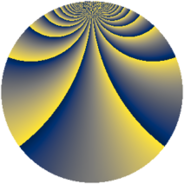# Properties

 Label 1815.2.bbLevel $1815$ Weight $2$ Character orbit 1815.bb Rep. character $\chi_{1815}(131,\cdot)$ Character field $\Q(\zeta_{22})$ Dimension $1760$ Sturm bound $528$

# Related objects

## Defining parameters

 Level: $$N$$ $$=$$ $$1815 = 3 \cdot 5 \cdot 11^{2}$$ Weight: $$k$$ $$=$$ $$2$$ Character orbit: $$[\chi]$$ $$=$$ 1815.bb (of order $$22$$ and degree $$10$$) Character conductor: $$\operatorname{cond}(\chi)$$ $$=$$ $$363$$ Character field: $$\Q(\zeta_{22})$$ Sturm bound: $$528$$

## Dimensions

The following table gives the dimensions of various subspaces of $$M_{2}(1815, [\chi])$$.

Total New Old
Modular forms 2680 1760 920
Cusp forms 2600 1760 840
Eisenstein series 80 0 80

## Trace form

 $$1760q + 4q^{3} - 176q^{4} - 8q^{9} + O(q^{10})$$ $$1760q + 4q^{3} - 176q^{4} - 8q^{9} - 54q^{12} - 18q^{15} - 144q^{16} + 8q^{22} + 88q^{24} + 176q^{25} - 20q^{27} + 44q^{31} - 4q^{33} + 40q^{34} + 4q^{36} - 8q^{37} + 28q^{42} + 72q^{48} + 140q^{49} + 66q^{51} - 44q^{52} + 154q^{54} - 8q^{55} + 154q^{57} - 104q^{58} - 12q^{60} - 512q^{64} - 2q^{66} - 88q^{67} + 40q^{69} - 24q^{70} + 44q^{73} - 4q^{75} - 396q^{76} - 84q^{78} - 44q^{79} - 32q^{81} + 136q^{82} + 132q^{85} - 412q^{88} - 88q^{90} + 132q^{91} - 26q^{93} + 40q^{97} - 56q^{99} + O(q^{100})$$

## Decomposition of $$S_{2}^{\mathrm{new}}(1815, [\chi])$$ into newform subspaces

The newforms in this space have not yet been added to the LMFDB.

## Decomposition of $$S_{2}^{\mathrm{old}}(1815, [\chi])$$ into lower level spaces

$$S_{2}^{\mathrm{old}}(1815, [\chi]) \cong$$ $$S_{2}^{\mathrm{new}}(363, [\chi])$$$$^{\oplus 2}$$